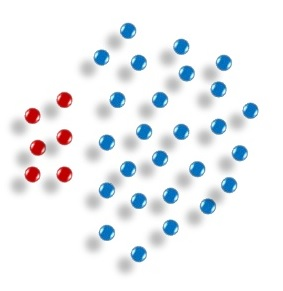## Imbalanced classification (or classification problems with low prevalence (low number of instances in one of the classes) can be challenging. In this post, I have discussed how we can model a problem with prevalence of 0.09% for positive class using gradient boosting and generalized linear model.

import numpy as np
import pandas as pd
import matplotlib.pyplot as plt
import matplotlib as mpl
from scipy import interp
from sklearn.preprocessing import scale
from sklearn.metrics import precision_score, recall_score, roc_auc_score, accuracy_score, roc_curve, auc, confusion_matrix
from sklearn.model_selection import cross_val_score, KFold, StratifiedKFold, train_test_split
from xgboost import XGBClassifier
import itertools
import glmnet
import xgboost as xgb
import shap
import seaborn as sns
sns.set_style("ticks")
mpl.rcParams['axes.linewidth'] = 3
mpl.rcParams['lines.linewidth'] =7
from IPython.core.display import display, HTML
display(HTML("<style>.container { width:95% !important; }</style>"))
import warnings
warnings.filterwarnings("ignore")
%matplotlib inline

# Unused Libraries here
# import keras
# from bayes_opt import BayesianOptimization
# from sklearn.model_selection import GridSearchCV, RandomizedSearchCV
# from fancyimpute import SoftImpute, IterativeImputer


# Functions

#*-*-*-*-*-*-*-*-*-*-*-*-*-*-*-*-*-*-*-*-*-*-*-*-*-*-*-*-*-*-*-*-*-*-*-*-*-*-*-*-*-*-*-*-*-*-
# Function: _x_y_maker

def _x_y_maker(df, target, to_drop):
"""
A function to receive the dataframe and make the X, and Y matrices:
----------
parameters

input: dataframe df
string target (class label)
list[string] to_drop (list of columns need to be dropped)

output: dataframe Feature matrix (df_X)
Target vector (Y)
"""
Targets = df[target]
to_drop.append(target)
Features = df.drop(to_drop, axis = 1)
Scaled_Features = scale(Features.values)
df_X = pd.DataFrame(data = Scaled_Features, columns = Features.columns.tolist())
Y = Targets.values

return df_X, Y

#*-*-*-*-*-*-*-*-*-*-*-*-*-*-*-*-*-*-*-*-*-*-*-*-*-*-*-*-*-*-*-*-*-*-*-*-*-*-*-*-*-*-*-*-*-*-
# Function: _plot_roc_nfolds_xgboost

def _plot_roc_nfolds_xgboost(df_X, Y,
n_folds = 10,
n_estimators = 100,
learning_rate = 0.05,
max_depth = 3,
min_child_weight = 5.0,
gamma = 0.5,
reg_alpha = 0.0,
reg_lambda = 1.0,
subsample = 0.9,
objective = "binary:logistic",
scale_pos_weight = 1.0,
shuffle = True,
random_state = 1367
):
"""
a function to plot k-fold cv ROC using xgboost
input parameters:
df_X : features : pandas dataframe (numpy array will be built inside the function)
Y : targets
n_folds : number of cv folds (default = 10)
n_estimators = number of trees (default = 100)
learning_rate : step size of xgboost (default = 0.05)
max_depth : maximum tree depth for xgboost (default = 3)
min_child_weight : (default = 5.0)
gamma : (default = 0.5)
reg_alpha : lasso penalty (L1) (default = 0.0)
reg_lambda : ridge penalty (L2) (default = 1.0)
subsample : subsample fraction (default = 0.9)
objective : objective function for ML (default = "binary:logistic" for classification)
scale_pos_weight : (default = 1.0)
shuffle : shuffle flag for cv (default = True)
random_state : (default = 1367)

"""

# Defining the data
X = scale(df_X.values)
y = Y
n_samples, n_features = X.shape

# Run classifier with cross-validation and plot ROC curves
cv = StratifiedKFold(n_splits = n_folds , shuffle = shuffle , random_state = random_state)
classifier = XGBClassifier(learning_rate = learning_rate,
n_estimators = n_estimators,
max_depth = max_depth,
min_child_weight = min_child_weight,
gamma = gamma,
reg_alpha = reg_alpha,
reg_lambda = reg_lambda,
subsample = subsample,
objective = objective,
scale_pos_weight = 1.,
base_score = np.mean(y),
seed = random_state,
random_state = random_state)

tprs = []
aucs = []
mean_fpr = np.linspace(0, 1, 100)
plt.figure(figsize=(18 , 13))
i = 0
for train, test in cv.split(X, y):
probas_ = classifier.fit(X[train], y[train]).predict_proba(X[test])
# Compute ROC curve and area the curve
fpr, tpr, thresholds = roc_curve(y[test], probas_[:, 1])
tprs.append(interp(mean_fpr, fpr, tpr))
tprs[-1] = 0.0
roc_auc = auc(fpr, tpr)
aucs.append(roc_auc)
plt.plot(fpr, tpr, lw=3, alpha=0.5,
label="ROC Fold %d (AUC = %0.2f)" % (i+1, roc_auc))

i += 1
plt.plot([0, 1], [0, 1], linestyle="--", lw=3, color="k",
label="Luck", alpha=.8)

mean_tpr = np.mean(tprs, axis=0)
mean_tpr[-1] = 1.0
mean_auc = auc(mean_fpr, mean_tpr)
std_auc = np.std(aucs)
plt.plot(mean_fpr, mean_tpr, color="navy",
label=r"Mean ROC (AUC = %0.2f $\pm$ %0.2f)" % (mean_auc, std_auc),
lw=4)

std_tpr = np.std(tprs, axis=0)
tprs_upper = np.minimum(mean_tpr + std_tpr, 1)
tprs_lower = np.maximum(mean_tpr - std_tpr, 0)
plt.fill_between(mean_fpr, tprs_lower, tprs_upper, color="grey", alpha=.4,
label=r"$\pm$ 1 Standard Deviation")

plt.xlim([-0.05, 1.05])
plt.ylim([-0.05, 1.05])
plt.xlabel("False Positive Rate" ,  fontweight = "bold" , fontsize=30)
plt.ylabel("True Positive Rate",fontweight = "bold" , fontsize=30)
plt.tick_params(axis="both", which="major", labelsize=20)
plt.legend( prop={"size":20} , loc = 4)
# plt.savefig("./roc_xgboost.pdf" ,bbox_inches="tight")
plt.show()

#*-*-*-*-*-*-*-*-*-*-*-*-*-*-*-*-*-*-*-*-*-*-*-*-*-*-*-*-*-*-*-*-*-*-*-*-*-*-*-*-*-*-*-*-*-*-
# Function: _permute

def _permute(df, random_state):
"""
a funtion to permute the rows of features and add them
to the dataframe as noisy features to explore stability
input parameters:
df : pandas dataframe
random_state : random seed for noisy features
"""
normal_df = df.copy().reset_index(drop = True)
noisy_df = df.copy()
noisy_df.rename(columns = {col : "noisy_" + col for col in noisy_df.columns}, inplace = True)
np.random.seed(seed = random_state)
noisy_df = noisy_df.reindex(np.random.permutation(noisy_df.index))
merged_df = pd.concat([normal_df, noisy_df.reset_index(drop = True)] , axis = 1)

return merged_df

#*-*-*-*-*-*-*-*-*-*-*-*-*-*-*-*-*-*-*-*-*-*-*-*-*-*-*-*-*-*-*-*-*-*-*-*-*-*-*-*-*-*-*-*-*-*-
# Function: _my_feature_selector

def _my_feature_selector(X, Y, n_iter = 1,
num_boost_round = 1000,
nfold = 10,
stratified = True,
metrics = ("auc"),
early_stopping_rounds = 50,
seed = 1367,
shuffle = True,
show_stdv = False,
params = None,
importance_type = "total_gain",
callbacks = False,
verbose_eval = False):
"""
a function to run xgboost kfolds cv and train the model based on the best boosting round of each iteration.
for different number of iterations. at each iteration noisy features are added as well. at each iteration
internal and external cv calculated.
NOTE: it is recommended to use ad-hoc parameters to make sure the model is under-fitted for the sake of feature selection
input parameters:
X: features (pandas dataframe or numpy array)
Y: targets (1D array or list)
n_iter: total number of iterations (default = 1)
num_boost_rounds: max number of boosting rounds, (default = 1000)
stratified: stratificaiton of the targets (default = True)
metrics: classification/regression metrics (default = ("auc))
early_stopping_rounds: the criteria for stopping if the test metric is not improved (default = 20)
seed: random seed (default = 1367)
shuffle: shuffling the data (default = True)
show_stdv = showing standard deviation of cv results (default = False)
params = set of parameters for xgboost cv
(default_params = {
"eval_metric" : "auc",
"tree_method": "hist",
"objective" : "binary:logistic",
"learning_rate" : 0.05,
"max_depth": 2,
"min_child_weight": 1,
"gamma" : 0.0,
"reg_alpha" : 0.0,
"reg_lambda" : 1.0,
"subsample" : 0.9,
"max_delta_step": 1,
"silent" : 1,
"scale_pos_weight" : 1
}
)
importance_type = importance type of xgboost as string (default = "total_gain")
the other options will be "weight", "gain", "cover", and "total_cover"
callbacks = printing callbacks for xgboost cv
(defaults = False, if True: [xgb.callback.print_evaluation(show_stdv = show_stdv),
xgb.callback.early_stop(early_stopping_rounds)])
verbose_eval : a flag to show the result during train on train/test sets (default = False)
outputs:
outputs_dict: a dict contains the fitted models, internal and external cv results for train/test sets
df_selected: a dataframe contains feature names with their frequency of being selected at each run of folds
"""
# callback flag
if(callbacks == True):
callbacks = [xgb.callback.print_evaluation(show_stdv = show_stdv),
xgb.callback.early_stop(early_stopping_rounds)]
else:
callbacks = None

# params
default_params = {
"eval_metric" : "auc",
"tree_method": "hist",
"objective" : "binary:logistic",
"learning_rate" : 0.05,
"max_depth": 2,
"min_child_weight": 1,
"gamma" : 0.0,
"reg_alpha" : 0.0,
"reg_lambda" : 1.0,
"subsample" : 0.9,
"max_delta_step": 1,
"silent" : 1,
"scale_pos_weight" : 1,
"base_score" : np.mean(Y)
}
# updating the default parameters with the input
if params is not None:
for key, val in params.items():
default_params[key] = val

# total results list
int_cv_train = []
int_cv_test = []
ext_cv_train = []
ext_cv_test = []
bst_list = []

# main loop
for iteration in range(n_iter):

# results list at iteration
int_cv_train2 = []
int_cv_test2 = []
ext_cv_train2 = []
ext_cv_test2 = []

# update random state
random_state = seed * iteration

X_permuted = _permute(df = X, random_state = random_state)
cols = X_permuted.columns.tolist()
Xval = X_permuted.values

# building DMatrix for training/testing + kfolds cv
cv = StratifiedKFold(n_splits = nfold , shuffle = shuffle , random_state = random_state)

for train_index, test_index in cv.split(Xval, Y):
Xval_train = Xval[train_index]
Xval_test = Xval[test_index]
Y_train = Y[train_index]
Y_test = Y[test_index]

X_train = pd.DataFrame(data = Xval_train, columns = cols)
X_test = pd.DataFrame(data = Xval_test, columns = cols)

dtrain = xgb.DMatrix(data = X_train, label = Y_train)
dtest = xgb.DMatrix(data = X_test, label = Y_test)

# watchlist during final training
watchlist = [(dtrain,"train"), (dtest,"eval")]

# a dict to store training results
evals_result = {}

# xgb cv
cv_results = xgb.cv(params = default_params,
dtrain = dtrain,
num_boost_round = num_boost_round,
nfold = nfold,
stratified = stratified,
metrics = metrics,
early_stopping_rounds = early_stopping_rounds,
seed = random_state,
verbose_eval = verbose_eval,
shuffle = shuffle,
callbacks = callbacks)
int_cv_train.append(cv_results.iloc[-1])
int_cv_test.append(cv_results.iloc[-1])
int_cv_train2.append(cv_results.iloc[-1])
int_cv_test2.append(cv_results.iloc[-1])

# xgb train
bst = xgb.train(params = default_params,
dtrain = dtrain,
num_boost_round = len(cv_results) - 1,
evals = watchlist,
evals_result = evals_result,
verbose_eval = verbose_eval)
# appending outputs
bst_list.append(bst)
ext_cv_train.append(evals_result["train"]["auc"][-1])
ext_cv_test.append(evals_result["eval"]["auc"][-1])
ext_cv_train2.append(evals_result["train"]["auc"][-1])
ext_cv_test2.append(evals_result["eval"]["auc"][-1])

print(F"-*-*-*-*-*-*-*-*- Iteration {iteration + 1} -*-*-*-*-*-*-*-*")
print(F"-*-*- Internal-{nfold} Folds-CV-Train = {np.mean(int_cv_train2):.3} +/- {np.std(int_cv_train2):.3} -*-*- Internal-{nfold} Folds-CV-Test = {np.mean(int_cv_test2):.3} +/- {np.std(int_cv_test2):.3} -*-*")
print(F"-*-*- External-{nfold} Folds-CV-Train = {np.mean(ext_cv_train2):.3} +/- {np.std(ext_cv_train2):.3} -*-*- External-{nfold} Folds-CV-Test = {np.mean(ext_cv_test2):.3} +/- {np.std(ext_cv_test2):.3} -*-*")

# putting together the outputs in one dict
outputs = {}
outputs["bst"] = bst_list
outputs["int_cv_train"] = int_cv_train
outputs["int_cv_test"] = int_cv_test
outputs["ext_cv_train"] = ext_cv_train
outputs["ext_cv_test"] = ext_cv_test

pruned_features = []
for bst_ in outputs["bst"]:
features_gain = bst_.get_score(importance_type = importance_type)
for key, value in features_gain.items():
pruned_features.append(key)

unique_elements, counts_elements = np.unique(pruned_features, return_counts = True)
counts_elements = [float(i) for i in list(counts_elements)]
df_features = pd.DataFrame(data = {"columns" : list(unique_elements) , "count" : counts_elements})
df_features.sort_values(by = ["count"], ascending = False, inplace = True)

# plotting
plt.figure()
df_features.sort_values(by = ["count"]).plot.barh(x = "columns", y = "count", color = "pink" , figsize = (8,8))
plt.show()

plt.figure(figsize = (16,12))

plt.subplot(2,2,1)
plt.title(F"Internal {nfold} CV {metrics.upper()} - Train", fontsize = 20)
plt.hist(outputs["int_cv_train"], bins = 20, color = "lightgreen")

plt.subplot(2,2,2)
plt.title(F"Internal {nfold} CV {metrics.upper()} - Test", fontsize = 20)
plt.hist(outputs["int_cv_test"], bins = 20, color = "lightgreen")

plt.subplot(2,2,3)
plt.title(F"External {nfold} CV {metrics.upper()} - Train", fontsize = 20)
plt.hist(outputs["ext_cv_train"], bins = 20, color = "lightblue")

plt.subplot(2,2,4)
plt.title(F"External {nfold} CV {metrics.upper()} - Test", fontsize = 20)
plt.hist(outputs["ext_cv_test"], bins = 20, color = "lightblue")

plt.show()

return outputs, df_features
#*-*-*-*-*-*-*-*-*-*-*-*-*-*-*-*-*-*-*-*-*-*-*-*-*-*-*-*-*-*-*-*-*-*-*-*-*-*-*-*-*-*-*-*-*-*-
# Function: Confidence Interval calculator for 95% significance

def _ConfidenceInterval(score, n):
"""
confidence interval for 95% significance level
input: score such as accuracy, auc
the size of test set n
"""

CI_range = 1.96 * np.sqrt( (score * (1 - score)) / n)
CI_pos = score + CI_range
CI_neg = score - CI_range
if(CI_neg < 0):
CI_neg = 0

return CI_neg, CI_pos

#*-*-*-*-*-*-*-*-*-*-*-*-*-*-*-*-*-*-*-*-*-*-*-*-*-*-*-*-*-*-*-*-*-*-*-*-*-*-*-*-*-*-*-*-*-*-
# Function: _bst_shap

def _bst_shap(X, Y, num_boost_round = 1000,
nfold = 10,
stratified = True,
metrics = ("auc"),
early_stopping_rounds = 50,
seed = 1367,
shuffle = True,
show_stdv = False,
params = None,
callbacks = False):
"""
a function to run xgboost kfolds cv with the params and train the model based on best boosting rounds
input parameters:
X: features
Y: targets
num_boost_rounds: max number of boosting rounds, (default = 1000)
stratified: stratificaiton of the targets (default = True)
metrics: classification/regression metrics (default = ("auc))
early_stopping_rounds: the criteria for stopping if the test metric is not improved (default = 20)
seed: random seed (default = 1367)
shuffle: shuffling the data (default = True)
show_stdv = showing standard deviation of cv results (default = False)
params = set of parameters for xgboost cv ( default_params = {
"eval_metric" : "auc",
"tree_method": "hist",
"objective" : "binary:logistic",
"learning_rate" : 0.05,
"max_depth": 2,
"min_child_weight": 5,
"gamma" : 0.0,
"reg_alpha" : 0.0,
"reg_lambda" : 1.0,
"subsample" : 0.9,
"silent" : 1,
"scale_pos_weight" : 1
}
)
callbacks = printing callbacks for xgboost cv (defaults: [xgb.callback.print_evaluation(show_stdv = show_stdv),
xgb.callback.early_stop(early_stopping_rounds)])
outputs:
bst: the trained model based on optimum number of boosting rounds
cv_results: a dataframe contains the cv-results (train/test + std of train/test) for metric
"""
# callback flag
if(callbacks == True):
callbacks = [xgb.callback.print_evaluation(show_stdv = show_stdv),
xgb.callback.early_stop(early_stopping_rounds)]
else:
callbacks = None

# params
default_params = {
"eval_metric" : "auc",
"tree_method": "hist",
"objective" : "binary:logistic",
"learning_rate" : 0.05,
"max_depth": 2,
"min_child_weight": 1,
"gamma" : 0.0,
"reg_alpha" : 0.0,
"reg_lambda" : 1.0,
"subsample" : 0.9,
"max_delta_step": 1,
"silent" : 1,
}
# updating the default parameters with the input
if params is not None:
for key, val in params.items():
default_params[key] = val

# building DMatrix for training
dtrain = xgb.DMatrix(data = X, label = Y)
print("-*-*-*-*-*-*-* CV Started *-*-*-*-*-*-*-")
cv_results = xgb.cv(params = default_params,
dtrain = dtrain,
num_boost_round = num_boost_round,
nfold = nfold,
stratified = stratified,
metrics = metrics,
early_stopping_rounds = early_stopping_rounds,
seed = seed,
shuffle = shuffle,
callbacks = callbacks)
print(F"*-*- Boosting Round = {len(cv_results) - 1} -*-*- Train = {cv_results.iloc[-1]:.3} -*-*- Test = {cv_results.iloc[-1]:.3} -*-*")
print("-*-*-*-*-*-*-* Train Started *-*-*-*-*-*-*-")
bst = xgb.train(params = default_params,
dtrain = dtrain,
num_boost_round = len(cv_results) - 1,
)

return bst, cv_results

#*-*-*-*-*-*-*-*-*-*-*-*-*-*-*-*-*-*-*-*-*-*-*-*-*-*-*-*-*-*-*-*-*-*-*-*-*-*-*-*-*-*-*-*-*-*-
# Function: _plot_xgboost_cv_score

def _plot_xgboost_cv_score(cv_results):
"""
a function to plot train/test cv score for
"""

import matplotlib as mpl
mpl.rcParams['axes.linewidth'] = 3
mpl.rcParams['lines.linewidth'] = 2
plt.figure(figsize=(10,8))
plt.errorbar(range(cv_results.shape), cv_results["train-auc-mean"],
yerr=cv_results["train-auc-std"], fmt = "--", ecolor="lightgreen", c = "navy", label = "Train (9-Folds)")

plt.errorbar(range(cv_results.shape), cv_results["test-auc-mean"],
yerr=cv_results["test-auc-std"], fmt = "--", ecolor="lightblue", c = "red", label = "Test (1-Fold)")

plt.xlabel("# of Boosting Rounds" ,  fontweight = "bold" , fontsize=30)
plt.ylabel("AUC",fontweight = "bold" , fontsize=30)
plt.tick_params(axis='both', which='major', labelsize=20)
plt.legend(loc = 4, prop={'size': 20})
plt.show()

#*-*-*-*-*-*-*-*-*-*-*-*-*-*-*-*-*-*-*-*-*-*-*-*-*-*-*-*-*-*-*-*-*-*-*-*-*-*-*-*-*-*-*-*-*-*-
# Function: _plot_importance

def _plot_importance(bst, importance_type = "total_gain", color = "pink", figsize = (10,10)):
"""
a function to plot feature importance in xgboost
"""
from xgboost import plot_importance
from pylab import rcParams
rcParams['figure.figsize'] = figsize
plot_importance(bst, importance_type = importance_type, color = color, xlabel = importance_type)
plt.show()

#*-*-*-*-*-*-*-*-*-*-*-*-*-*-*-*-*-*-*-*-*-*-*-*-*-*-*-*-*-*-*-*-*-*-*-*-*-*-*-*-*-*-*-*-*-*-
# Function: _plot_confusion_matrix
def _plot_confusion_matrix(cm, classes,
normalize=False,
title='Confusion matrix',
cmap=plt.cm.Greens):
"""
This function prints and plots the confusion matrix.
Normalization can be applied by setting normalize=True.
"""
if normalize:
cm = cm.astype('float') / cm.sum(axis=1)[:, np.newaxis]
print("Normalized confusion matrix")
else:
print('Confusion matrix, without normalization')

print(cm)

plt.imshow(cm, interpolation='nearest', cmap=cmap)
plt.title(title, fontsize = 20)
plt.colorbar()
tick_marks = np.arange(len(classes))
plt.xticks(tick_marks, classes)
plt.yticks(tick_marks, classes)

fmt = '.2f' if normalize else 'd'
thresh = cm.max() / 2.
for i, j in itertools.product(range(cm.shape), range(cm.shape)):
plt.text(j, i, format(cm[i, j], fmt),
horizontalalignment="center",
color="white" if cm[i, j] > thresh else "black")

plt.ylabel('True label', fontsize = 20)
plt.xlabel('Predicted label', fontsize = 20)
plt.tick_params(axis='both', which='major', labelsize=20)
plt.tight_layout()

#*-*-*-*-*-*-*-*-*-*-*-*-*-*-*-*-*-*-*-*-*-*-*-*-*-*-*-*-*-*-*-*-*-*-*-*-*-*-*-*-*-*-*-*-*-*-
# Function: _clf_xgboost

def _clf_xgboost(df_X, Y, test_size, n_folds):
X = df_X.values
clf = XGBClassifier(learning_rate = 0.05,
n_estimators = 100,
max_depth = 3,
min_child_weight = 5.,
# max_delta_step= 1,
gamma = 0.5,
reg_alpha = 0.0,
reg_lambda = 1.0,
subsample = 0.9,
colsample_bytree = 0.9,
objective = "binary:logistic",
#scale_pos_weight = (len(Y) - sum(Y)) / sum(Y),
base_score = np.mean(Y),
seed = 1367,
random_state = 1367)

X_train, X_test, y_train, y_test = train_test_split(df_X, Y, test_size = test_size, shuffle = True, random_state = 1367, stratify = Y)
clf.fit(X_train, y_train, eval_metric="auc", early_stopping_rounds = 50, verbose = False, eval_set=[(X_test, y_test)])
accuracy_score = clf.score(X_test, y_test)
cv_roc_score = cross_val_score(clf, X, Y, cv = n_folds, scoring = "roc_auc")
y_pred_prob = clf.predict_proba(X_test)
roc_score = roc_auc_score(y_test, y_pred_prob[:,1])
fpr, tpr, thresholds = roc_curve(y_test, y_pred_prob[:,1])
optimal_idx = np.argmax(np.abs(tpr - fpr))
optimal_threshold = thresholds[optimal_idx]

return accuracy_score, roc_score, cv_roc_score, y_pred_prob, X_test, y_test, optimal_threshold

#*-*-*-*-*-*-*-*-*-*-*-*-*-*-*-*-*-*-*-*-*-*-*-*-*-*-*-*-*-*-*-*-*-*-*-*-*-*-*-*-*-*-*-*-*-*-
# Function: _xgboost_auc_hist
def _xgboost_auc_hist(df_X, Y, n_iterations = 100, alpha = 0.95):
"""
plot histogram of ROC AUC for N stratified classification for 95% significance level
"""

n_size = int(len(Y) * 0.30)

# Main Loop
scores = list()
for i in range(n_iterations):
# split the data into train/test sets
X_train, X_test, y_train, y_test = train_test_split(df_X, Y, test_size = n_size, shuffle = True, random_state = 1367 * i , stratify = Y)
# fit the xgboost classifier
clf = XGBClassifier(learning_rate = 0.05,
n_estimators = 100,
max_depth = 3,
min_child_weight = 5.,
# max_delta_step= 1,
gamma = 0.5,
reg_alpha = 0.0,
reg_lambda = 1.0,
subsample = 0.9,
colsample_bytree = 0.9,
objective = "binary:logistic",
#scale_pos_weight = (len(Y) - sum(Y)) / sum(Y),
base_score = np.mean(Y),
seed = 1367 * i ,
random_state = 1367 * i)
clf.fit(X_train, y_train, eval_metric = "auc", early_stopping_rounds = 50, verbose = False, eval_set = [(X_test, y_test)])
# evaluate model
predictions = clf.predict_proba(X_test)[:,1]
pred_auc = roc_auc_score(y_test, predictions)
scores.append(pred_auc)

# Ploting the scores
plt.figure(figsize=(10,5))
plt.hist(scores, color = "pink")
plt.xlabel("ROC AUC", fontsize = 30)
plt.tick_params(axis = "both", which = "major", labelsize = 15)
plt.show()

# confidence intervals
alpha = 0.95
p = ((1.0 - alpha)/2.0) * 100
lower = max(0.0, np.percentile(scores, p))
p = (alpha + ((1.0 - alpha)/2.0)) * 100
upper = min(1.0, np.percentile(scores, p))
print(F"{alpha*100}% Significance Level - ROC AUC Confidence Interval= [{lower:.3f} , {upper:.3f}]")

#*-*-*-*-*-*-*-*-*-*-*-*-*-*-*-*-*-*-*-*-*-*-*-*-*-*-*-*-*-*-*-*-*-*-*-*-*-*-*-*-*-*-*-*-*-*-
# Function: _glmnet

def _glmnet(X, Y, alpha = 0.1, n_splits = 10, scoring = "roc_auc"):
"""
a function for standard glmnet
input parameters: pandas dataframe X
class labels Y
alpha = 0.1
n_splits = 10
scoring = "roc_auc"
output: glmnet model

"""
model = glmnet.LogitNet(alpha = alpha,
n_lambda = 100,
n_splits = n_splits,
cut_point = 1.0,
scoring = scoring,
n_jobs = -1,
random_state = 1367)
model.fit(X, Y)

return model

#*-*-*-*-*-*-*-*-*-*-*-*-*-*-*-*-*-*-*-*-*-*-*-*-*-*-*-*-*-*-*-*-*-*-*-*-*-*-*-*-*-*-*-*-*-*-
# Function: _plot_glmnet_cv_score

def _plot_glmnet_cv_score(model):
"""
a function to plot cv scores vs lambda
parameters:
model : a fitted glmnet object
"""
mpl.rcParams['axes.linewidth'] = 3
mpl.rcParams['lines.linewidth'] =2
plt.figure(figsize=(10,6))
plt.errorbar(-np.log(model.lambda_path_), model.cv_mean_score_, yerr=model.cv_standard_error_ , c = "r", ecolor="k", marker = "o" )
plt.vlines(-np.log(model.lambda_best_), ymin = min(model.cv_mean_score_) - 0.05 , ymax = max(model.cv_mean_score_) + 0.05, lw = 3, linestyles = "--", colors = "b" ,label = "best $\lambda$")
plt.vlines(-np.log(model.lambda_max_), ymin = min(model.cv_mean_score_) - 0.05 , ymax = max(model.cv_mean_score_) + 0.05, lw = 3, linestyles = "--", colors = "c" ,label = "max $\lambda$")
plt.tick_params(axis='both', which='major', labelsize = 12)
plt.grid(True)
plt.ylim([min(model.cv_mean_score_) - 0.05, max(model.cv_mean_score_) + 0.05])
plt.legend(loc = 4, prop={'size': 20})
plt.xlabel("$-Log(\lambda)$" , fontsize = 20)
plt.ylabel(F"Mean {model.n_splits} Folds CV {(model.scoring).upper()}", fontsize = 20)
plt.title(F"Best $\lambda$ = {model.lambda_best_:.2} with {len(np.nonzero(  model.coef_))} Features" , fontsize = 20)
plt.show()

#*-*-*-*-*-*-*-*-*-*-*-*-*-*-*-*-*-*-*-*-*-*-*-*-*-*-*-*-*-*-*-*-*-*-*-*-*-*-*-*-*-*-*-*-*-*-
# Function: _plot_glmnet_coeff_path

def _plot_glmnet_coeff_path(model, df):
"""
a function to plot coefficients vs lambda
parameters:
model : a fitted glmnet object
df: in case that the input is the pandas dataframe,
the column names of the coeff. will appear as a legend
"""
mpl.rcParams['axes.linewidth'] = 3
mpl.rcParams['lines.linewidth'] =2
plt.figure(figsize=(10,6))
if not df.empty:
for i in list(np.nonzero(np.reshape(model.coef_, (1,-1)))):
plt.plot(-np.log(model.lambda_path_) ,(model.coef_path_.reshape(-1,model.coef_path_.shape[-1]))[i,:], label = df.columns.values.tolist()[i]);
plt.legend(loc= "right", bbox_to_anchor=(1.2 , .5), ncol=1, fancybox=True, shadow=True)

else:
for i in list(np.nonzero(np.reshape(model.coef_, (1,-1)))):
plt.plot(-np.log(model.lambda_path_) ,(model.coef_path_.reshape(-1, model.coef_path_.shape[-1]))[i,:]);

plt.tick_params(axis='both', which='major', labelsize = 12)
plt.ylabel("Coefficients", fontsize = 20)
plt.xlabel("-$Log(\lambda)$", fontsize = 20)
plt.title(F"Best $\lambda$ = {model.lambda_best_:.2} with {len(np.nonzero(model.coef_))} Features" , fontsize = 20)
plt.grid(True)
plt.show()

#*-*-*-*-*-*-*-*-*-*-*-*-*-*-*-*-*-*-*-*-*-*-*-*-*-*-*-*-*-*-*-*-*-*-*-*-*-*-*-*-*-*-*-*-*-*-
# Function: _df_glmnet_coeff_path

def _df_glmnet_coeff_path(model, df):
"""
a function to build a dataframe for nonzero coeff.
parameters:
model : a fitted glmnet object
df: in case that the input is the pandas dataframe,
the column names of the coeff. will appear as a legend

"""
idx = list(np.nonzero(np.reshape(model.coef_, (1,-1))))
dct = dict( zip([df.columns.tolist()[i] for i in idx], [model.coef_[i] for i in idx]))

return pd.DataFrame(data = dct.items() , columns = ["Features", "Coeffs"]).sort_values(by = "Coeffs", ascending = False).reset_index(drop = True)

#*-*-*-*-*-*-*-*-*-*-*-*-*-*-*-*-*-*-*-*-*-*-*-*-*-*-*-*-*-*-*-*-*-*-*-*-*-*-*-*-*-*-*-*-*-*-
# Function: _glmnet_best_score_alpha

def _glmnet_best_score_alpha(X, Y):
"""
a function to run glmnet for different alpha
"""

def logitnet_nonzero_coef(g):
idx = np.where(g.coef_ != 0)
return pd.DataFrame({"idx": idx, "coef": g.coef_[idx]})

alpha_list = np.arange(0.0, 0.5, 0.05)
mean_auc = []
std_auc = []
n_features = []
for alpha in alpha_list:
model = _glmnet(X, Y, alpha = alpha)
mean_auc.append(model.cv_mean_score_[model.lambda_best_inx_])
std_auc.append(model.cv_standard_error_[model.lambda_best_inx_])
fe = logitnet_nonzero_coef(model)
n_features.append(fe.shape)

mpl.rcParams['axes.linewidth'] = 3
mpl.rcParams['lines.linewidth'] =2
plt.figure(figsize=(12,6))

plt.subplot(1,2,1)
plt.errorbar(alpha_list, mean_auc, yerr = std_auc, c = "r", ecolor="k", marker = "o" )
plt.tick_params(axis='both', which='major', labelsize = 12)
plt.xlabel(r"$\alpha$" , fontsize = 20)
plt.ylabel("Mean 10 Folds CV AUC", fontsize = 20)
plt.grid(True)

plt.subplot(1,2,2)
plt.plot(alpha_list, n_features, c = "r",  marker = "o" )
plt.tick_params(axis='both', which='major', labelsize = 12)
plt.xlabel(r"$\alpha$" , fontsize = 20)
plt.ylabel("Number of Features", fontsize = 20)
plt.grid(True)

plt.show()



### First, I loaded the data into a pandas dataframe to get some idea.

# readin the data into a dataframe
dateparser = lambda x: pd.datetime.strptime(x, "%Y-%m-%d")
parse_dates = ["date"],
date_parser = dateparser,
encoding = "cp1252")

print("Shape: {}".format(df_raw.shape))
print("Prevalence = {:.3f}%".format(df_raw["failure"].sum()/df_raw.shape * 100))

Shape: (124494, 12)
Prevalence = 0.085%

df_raw.head()

date device failure attribute1 attribute2 attribute3 attribute4 attribute5 attribute6 attribute7 attribute8 attribute9
0 2015-01-01 S1F01085 0 215630672 56 0 52 6 407438 0 0 7
1 2015-01-01 S1F0166B 0 61370680 0 3 0 6 403174 0 0 0
2 2015-01-01 S1F01E6Y 0 173295968 0 0 0 12 237394 0 0 0
3 2015-01-01 S1F01JE0 0 79694024 0 0 0 6 410186 0 0 0
4 2015-01-01 S1F01R2B 0 135970480 0 0 0 15 313173 0 0 3

### Printing out the statistical description of the data. It would help us to have idea about the variation of the each column in the data.

df_raw.describe()

failure attribute1 attribute2 attribute3 attribute4 attribute5 attribute6 attribute7 attribute8 attribute9
count 124494.000000 1.244940e+05 124494.000000 124494.000000 124494.000000 124494.000000 124494.000000 124494.000000 124494.000000 124494.000000
mean 0.000851 1.223881e+08 159.484762 9.940455 1.741120 14.222669 260172.657726 0.292528 0.292528 12.451524
std 0.029167 7.045933e+07 2179.657730 185.747321 22.908507 15.943028 99151.078547 7.436924 7.436924 191.425623
min 0.000000 0.000000e+00 0.000000 0.000000 0.000000 1.000000 8.000000 0.000000 0.000000 0.000000
25% 0.000000 6.128476e+07 0.000000 0.000000 0.000000 8.000000 221452.000000 0.000000 0.000000 0.000000
50% 0.000000 1.227974e+08 0.000000 0.000000 0.000000 10.000000 249799.500000 0.000000 0.000000 0.000000
75% 0.000000 1.833096e+08 0.000000 0.000000 0.000000 12.000000 310266.000000 0.000000 0.000000 0.000000
max 1.000000 2.441405e+08 64968.000000 24929.000000 1666.000000 98.000000 689161.000000 832.000000 832.000000 18701.000000

### Printing out the number of Null values in each column. These numbers should be imputed before feeding into the classifier.

df_raw.isnull().sum()

date          0
device        0
failure       0
attribute1    0
attribute2    0
attribute3    0
attribute4    0
attribute5    0
attribute6    0
attribute7    0
attribute8    0
attribute9    0
dtype: int64


### Checking the data types of the columns to make sure all the columns have continuous values as it is mentioned in the meta data, except date and device

df_raw.dtypes

date          datetime64[ns]
device                object
failure                int64
attribute1             int64
attribute2             int64
attribute3             int64
attribute4             int64
attribute5             int64
attribute6             int64
attribute7             int64
attribute8             int64
attribute9             int64
dtype: object


### methods = {‘pearson’, ‘kendall’, ‘spearman’}

df_raw.corr(method = "kendall")

failure attribute1 attribute2 attribute3 attribute4 attribute5 attribute6 attribute7 attribute8 attribute9
failure 1.000000 0.001611 0.053361 0.003208 0.057295 0.003475 0.002337 0.096926 0.096926 0.004633
attribute1 0.001611 1.000000 -0.001004 0.001960 0.001289 -0.003713 -0.001779 -0.002030 -0.002030 -0.002662
attribute2 0.053361 -0.001004 1.000000 -0.018322 0.219415 -0.022125 -0.062126 0.107491 0.107491 -0.027759
attribute3 0.003208 0.001960 -0.018322 1.000000 0.117459 0.089091 0.056736 -0.009622 -0.009622 0.369092
attribute4 0.057295 0.001289 0.219415 0.117459 1.000000 -0.017980 0.009706 0.160216 0.160216 0.046189
attribute5 0.003475 -0.003713 -0.022125 0.089091 -0.017980 1.000000 0.057295 -0.016603 -0.016603 0.026998
attribute6 0.002337 -0.001779 -0.062126 0.056736 0.009706 0.057295 1.000000 -0.013186 -0.013186 0.069585
attribute7 0.096926 -0.002030 0.107491 -0.009622 0.160216 -0.016603 -0.013186 1.000000 1.000000 -0.017097
attribute8 0.096926 -0.002030 0.107491 -0.009622 0.160216 -0.016603 -0.013186 1.000000 1.000000 -0.017097
attribute9 0.004633 -0.002662 -0.027759 0.369092 0.046189 0.026998 0.069585 -0.017097 -0.017097 1.000000
# Number of unique devices: this can be used as feauture based on the demand
df_raw["device"].nunique()

1169


### Preprocessing: attribute7 and attribute8 are totally correlated (the same feature). For now, I am gonna drop date and device as well. Device has 1169 unique values that can be used as features.

df = df_raw.copy()
target = "failure"
to_drop = ["date", "device", "attribute8"]
df_X, Y = _x_y_maker(df, target, to_drop)


### Printing the first 5 rows of the sclaed features.

df_X.head()

attribute1 attribute2 attribute3 attribute4 attribute5 attribute6 attribute7 attribute9
0 1.323358 -0.047478 -0.053516 2.193905 -0.515755 1.485268 -0.039335 -0.028479
1 -0.865998 -0.073170 -0.037365 -0.076004 -0.515755 1.442263 -0.039335 -0.065047
2 0.722517 -0.073170 -0.053516 -0.076004 -0.139414 -0.229738 -0.039335 -0.065047
3 -0.605942 -0.073170 -0.053516 -0.076004 -0.515755 1.512983 -0.039335 -0.065047
4 0.192770 -0.073170 -0.053516 -0.076004 0.048757 0.534543 -0.039335 -0.049375

### Checking the correlation again.

df_X.corr()

attribute1 attribute2 attribute3 attribute4 attribute5 attribute6 attribute7 attribute9
attribute1 1.000000 -0.004250 0.003701 0.001836 -0.003376 -0.001522 0.000151 0.001121
attribute2 -0.004250 1.000000 -0.002617 0.146593 -0.013999 -0.026350 0.141367 -0.002736
attribute3 0.003701 -0.002617 1.000000 0.097452 -0.006696 0.009027 -0.001884 0.532366
attribute4 0.001836 0.146593 0.097452 1.000000 -0.009773 0.024870 0.045631 0.036069
attribute5 -0.003376 -0.013999 -0.006696 -0.009773 1.000000 -0.017049 -0.009384 0.005949
attribute6 -0.001522 -0.026350 0.009027 0.024870 -0.017049 1.000000 -0.012207 0.021152
attribute7 0.000151 0.141367 -0.001884 0.045631 -0.009384 -0.012207 1.000000 0.006861
attribute9 0.001121 -0.002736 0.532366 0.036069 0.005949 0.021152 0.006861 1.000000

### Running my feature selector along with noise with 10-folds CV for multiple iterations to make sure about the selected features. This would help to have a simpler model with the same performance

# choosing params to under-fit the model for feature selection + noisy features. This would help to have simpler model
params = {
"learning_rate" : 0.05,
"max_depth": 2,
"min_child_weight": 5,
"gamma" : 0.5

}
o_, d_ = _my_feature_selector(df_X, Y, n_iter = 10,
params = params,
seed = 1367,
callbacks = False)

-*-*-*-*-*-*-*-*- Iteration 1 -*-*-*-*-*-*-*-*
-*-*- Internal-10 Folds-CV-Train = 0.935 +/- 0.00485 -*-*- Internal-10 Folds-CV-Test = 0.897 +/- 0.00849 -*-*
-*-*- External-10 Folds-CV-Train = 0.933 +/- 0.005 -*-*- External-10 Folds-CV-Test = 0.898 +/- 0.0435 -*-*
-*-*-*-*-*-*-*-*- Iteration 2 -*-*-*-*-*-*-*-*
-*-*- Internal-10 Folds-CV-Train = 0.932 +/- 0.00891 -*-*- Internal-10 Folds-CV-Test = 0.896 +/- 0.00948 -*-*
-*-*- External-10 Folds-CV-Train = 0.931 +/- 0.00924 -*-*- External-10 Folds-CV-Test = 0.892 +/- 0.0413 -*-*
-*-*-*-*-*-*-*-*- Iteration 3 -*-*-*-*-*-*-*-*
-*-*- Internal-10 Folds-CV-Train = 0.934 +/- 0.0065 -*-*- Internal-10 Folds-CV-Test = 0.896 +/- 0.00591 -*-*
-*-*- External-10 Folds-CV-Train = 0.933 +/- 0.00634 -*-*- External-10 Folds-CV-Test = 0.892 +/- 0.0571 -*-*
-*-*-*-*-*-*-*-*- Iteration 4 -*-*-*-*-*-*-*-*
-*-*- Internal-10 Folds-CV-Train = 0.935 +/- 0.00669 -*-*- Internal-10 Folds-CV-Test = 0.897 +/- 0.00905 -*-*
-*-*- External-10 Folds-CV-Train = 0.933 +/- 0.00776 -*-*- External-10 Folds-CV-Test = 0.894 +/- 0.0548 -*-*
-*-*-*-*-*-*-*-*- Iteration 5 -*-*-*-*-*-*-*-*
-*-*- Internal-10 Folds-CV-Train = 0.934 +/- 0.00684 -*-*- Internal-10 Folds-CV-Test = 0.897 +/- 0.00607 -*-*
-*-*- External-10 Folds-CV-Train = 0.932 +/- 0.00653 -*-*- External-10 Folds-CV-Test = 0.894 +/- 0.052 -*-*
-*-*-*-*-*-*-*-*- Iteration 6 -*-*-*-*-*-*-*-*
-*-*- Internal-10 Folds-CV-Train = 0.932 +/- 0.0068 -*-*- Internal-10 Folds-CV-Test = 0.898 +/- 0.0082 -*-*
-*-*- External-10 Folds-CV-Train = 0.931 +/- 0.00678 -*-*- External-10 Folds-CV-Test = 0.892 +/- 0.0493 -*-*
-*-*-*-*-*-*-*-*- Iteration 7 -*-*-*-*-*-*-*-*
-*-*- Internal-10 Folds-CV-Train = 0.931 +/- 0.0071 -*-*- Internal-10 Folds-CV-Test = 0.896 +/- 0.00643 -*-*
-*-*- External-10 Folds-CV-Train = 0.93 +/- 0.00858 -*-*- External-10 Folds-CV-Test = 0.892 +/- 0.0339 -*-*
-*-*-*-*-*-*-*-*- Iteration 8 -*-*-*-*-*-*-*-*
-*-*- Internal-10 Folds-CV-Train = 0.933 +/- 0.00951 -*-*- Internal-10 Folds-CV-Test = 0.898 +/- 0.00782 -*-*
-*-*- External-10 Folds-CV-Train = 0.932 +/- 0.00995 -*-*- External-10 Folds-CV-Test = 0.892 +/- 0.0766 -*-*
-*-*-*-*-*-*-*-*- Iteration 9 -*-*-*-*-*-*-*-*
-*-*- Internal-10 Folds-CV-Train = 0.929 +/- 0.00658 -*-*- Internal-10 Folds-CV-Test = 0.895 +/- 0.00973 -*-*
-*-*- External-10 Folds-CV-Train = 0.928 +/- 0.00671 -*-*- External-10 Folds-CV-Test = 0.896 +/- 0.0523 -*-*
-*-*-*-*-*-*-*-*- Iteration 10 -*-*-*-*-*-*-*-*
-*-*- Internal-10 Folds-CV-Train = 0.935 +/- 0.00538 -*-*- Internal-10 Folds-CV-Test = 0.898 +/- 0.00774 -*-*
-*-*- External-10 Folds-CV-Train = 0.933 +/- 0.0058 -*-*- External-10 Folds-CV-Test = 0.883 +/- 0.0507 -*-*

<Figure size 432x288 with 0 Axes>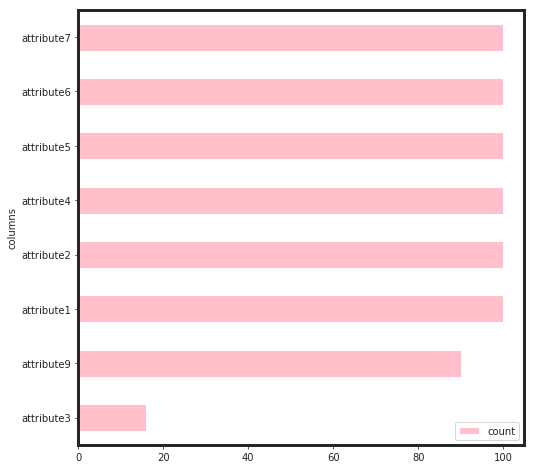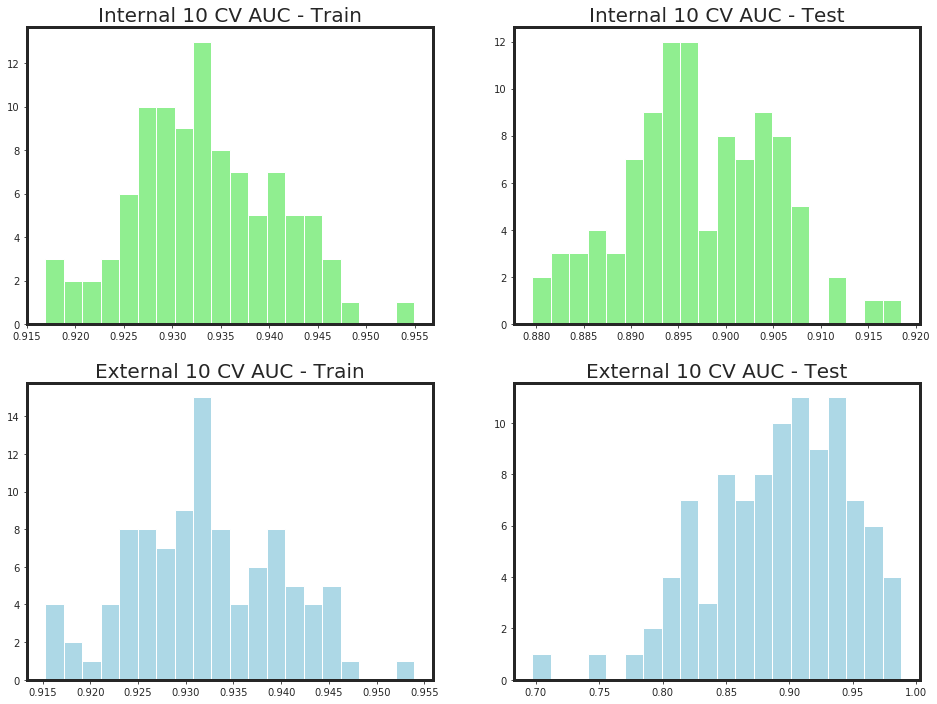params = {
"learning_rate" : 0.05,
"max_depth": 2,
"min_child_weight": 5.,
"gamma" : 0.5
}
bst, cvr = _bst_shap(df_X, Y, params = params, callbacks = True)

-*-*-*-*-*-*-* CV Started *-*-*-*-*-*-*-
[22:09:38] Tree method is selected to be 'hist', which uses a single updater grow_fast_histmaker.
[22:09:38] Tree method is selected to be 'hist', which uses a single updater grow_fast_histmaker.
[22:09:38] Tree method is selected to be 'hist', which uses a single updater grow_fast_histmaker.
[22:09:39] Tree method is selected to be 'hist', which uses a single updater grow_fast_histmaker.
[22:09:39] Tree method is selected to be 'hist', which uses a single updater grow_fast_histmaker.
[22:09:39] Tree method is selected to be 'hist', which uses a single updater grow_fast_histmaker.
[22:09:39] Tree method is selected to be 'hist', which uses a single updater grow_fast_histmaker.
[22:09:39] Tree method is selected to be 'hist', which uses a single updater grow_fast_histmaker.
[22:09:39] Tree method is selected to be 'hist', which uses a single updater grow_fast_histmaker.
[22:09:39] Tree method is selected to be 'hist', which uses a single updater grow_fast_histmaker.
	train-auc:0.5	test-auc:0.5
Multiple eval metrics have been passed: 'test-auc' will be used for early stopping.

Will train until test-auc hasn't improved in 50 rounds.
	train-auc:0.5	test-auc:0.5
	train-auc:0.5	test-auc:0.5
	train-auc:0.5	test-auc:0.5
	train-auc:0.5	test-auc:0.5
	train-auc:0.5	test-auc:0.5
	train-auc:0.5	test-auc:0.5
	train-auc:0.5	test-auc:0.5
	train-auc:0.5	test-auc:0.5
	train-auc:0.5	test-auc:0.5
	train-auc:0.5	test-auc:0.5
	train-auc:0.5	test-auc:0.5
	train-auc:0.5	test-auc:0.5
	train-auc:0.5	test-auc:0.5
	train-auc:0.5	test-auc:0.5
	train-auc:0.5	test-auc:0.5
	train-auc:0.5	test-auc:0.5
	train-auc:0.5	test-auc:0.5
	train-auc:0.502591	test-auc:0.499988
	train-auc:0.507799	test-auc:0.499972
	train-auc:0.515655	test-auc:0.499924
	train-auc:0.515655	test-auc:0.499924
	train-auc:0.521354	test-auc:0.504899
	train-auc:0.527633	test-auc:0.509397
	train-auc:0.544239	test-auc:0.51922
	train-auc:0.547894	test-auc:0.519192
	train-auc:0.562889	test-auc:0.528576
	train-auc:0.562888	test-auc:0.528573
	train-auc:0.575345	test-auc:0.551115
	train-auc:0.589815	test-auc:0.570037
	train-auc:0.589813	test-auc:0.570032
	train-auc:0.589815	test-auc:0.570038
	train-auc:0.594991	test-auc:0.579028
	train-auc:0.603815	test-auc:0.588018
	train-auc:0.607991	test-auc:0.587983
	train-auc:0.607994	test-auc:0.587977
	train-auc:0.609034	test-auc:0.587952
	train-auc:0.611603	test-auc:0.58792
	train-auc:0.611606	test-auc:0.58792
	train-auc:0.621348	test-auc:0.592728
	train-auc:0.630541	test-auc:0.59751
	train-auc:0.641352	test-auc:0.610898
	train-auc:0.645456	test-auc:0.619846
	train-auc:0.649023	test-auc:0.624774
	train-auc:0.649023	test-auc:0.629293
	train-auc:0.652603	test-auc:0.638277
	train-auc:0.656164	test-auc:0.638207
	train-auc:0.656163	test-auc:0.638208
	train-auc:0.660153	test-auc:0.638036
	train-auc:0.660154	test-auc:0.638033
	train-auc:0.668212	test-auc:0.637722
	train-auc:0.683198	test-auc:0.651949
	train-auc:0.68676	test-auc:0.65183
	train-auc:0.702173	test-auc:0.664918
	train-auc:0.705216	test-auc:0.673904
	train-auc:0.716932	test-auc:0.688166
	train-auc:0.746947	test-auc:0.705966
	train-auc:0.766238	test-auc:0.732417
	train-auc:0.76625	test-auc:0.732301
	train-auc:0.781952	test-auc:0.749171
	train-auc:0.785332	test-auc:0.753368
	train-auc:0.788794	test-auc:0.753104
	train-auc:0.788767	test-auc:0.753255
	train-auc:0.789759	test-auc:0.757569
	train-auc:0.789792	test-auc:0.757407
	train-auc:0.791717	test-auc:0.762459
	train-auc:0.791741	test-auc:0.762543
	train-auc:0.794139	test-auc:0.771179
	train-auc:0.79414	test-auc:0.771128
	train-auc:0.798393	test-auc:0.78537
	train-auc:0.799311	test-auc:0.790212
	train-auc:0.799362	test-auc:0.790179
	train-auc:0.799436	test-auc:0.790156
	train-auc:0.804061	test-auc:0.789724
	train-auc:0.809716	test-auc:0.793472
	train-auc:0.81023	test-auc:0.793571
	train-auc:0.810291	test-auc:0.793676
	train-auc:0.810273	test-auc:0.794129
	train-auc:0.815368	test-auc:0.793779
	train-auc:0.818557	test-auc:0.798271
	train-auc:0.818553	test-auc:0.798253
	train-auc:0.818556	test-auc:0.798244
	train-auc:0.819048	test-auc:0.798204
	train-auc:0.819046	test-auc:0.798256
	train-auc:0.819084	test-auc:0.798343
	train-auc:0.819094	test-auc:0.798331
	train-auc:0.826427	test-auc:0.810713
	train-auc:0.827297	test-auc:0.815134
	train-auc:0.827317	test-auc:0.820203
	train-auc:0.82735	test-auc:0.820224
	train-auc:0.835594	test-auc:0.836472
	train-auc:0.839335	test-auc:0.83642
	train-auc:0.839334	test-auc:0.836343
	train-auc:0.844798	test-auc:0.840614
	train-auc:0.845237	test-auc:0.840554
	train-auc:0.845206	test-auc:0.840589
	train-auc:0.845202	test-auc:0.840541
	train-auc:0.845634	test-auc:0.840411
	train-auc:0.846109	test-auc:0.840341
	train-auc:0.8461	test-auc:0.840213
	train-auc:0.846579	test-auc:0.840174
	train-auc:0.847455	test-auc:0.840033
	train-auc:0.848847	test-auc:0.839937
	train-auc:0.848889	test-auc:0.839934
	train-auc:0.851638	test-auc:0.839058
	train-auc:0.851658	test-auc:0.839094
	train-auc:0.851696	test-auc:0.839025
	train-auc:0.851761	test-auc:0.839107
	train-auc:0.855548	test-auc:0.838203
	train-auc:0.855952	test-auc:0.838216
	train-auc:0.859843	test-auc:0.837271
	train-auc:0.859896	test-auc:0.837218
	train-auc:0.862772	test-auc:0.844197
	train-auc:0.866681	test-auc:0.847177
	train-auc:0.866765	test-auc:0.847206
	train-auc:0.867214	test-auc:0.847115
	train-auc:0.868626	test-auc:0.855585
	train-auc:0.869564	test-auc:0.859661
	train-auc:0.869588	test-auc:0.859604
	train-auc:0.870008	test-auc:0.859496
	train-auc:0.870083	test-auc:0.859439
	train-auc:0.870515	test-auc:0.859276
	train-auc:0.871057	test-auc:0.859007
	train-auc:0.871131	test-auc:0.859277
	train-auc:0.871211	test-auc:0.859541
	train-auc:0.871218	test-auc:0.859699
	train-auc:0.871588	test-auc:0.863687
	train-auc:0.871767	test-auc:0.863805
	train-auc:0.87185	test-auc:0.864008
	train-auc:0.871903	test-auc:0.863906
	train-auc:0.871946	test-auc:0.863755
	train-auc:0.871992	test-auc:0.863533
	train-auc:0.87198	test-auc:0.863675
	train-auc:0.872139	test-auc:0.863535
	train-auc:0.872082	test-auc:0.86365
	train-auc:0.872188	test-auc:0.863516
	train-auc:0.872232	test-auc:0.86354
	train-auc:0.872228	test-auc:0.863652
	train-auc:0.872266	test-auc:0.863696
	train-auc:0.872255	test-auc:0.863683
	train-auc:0.872303	test-auc:0.863636
	train-auc:0.872336	test-auc:0.863547
	train-auc:0.872402	test-auc:0.863523
	train-auc:0.872441	test-auc:0.863512
	train-auc:0.876281	test-auc:0.865899
	train-auc:0.876307	test-auc:0.865908
	train-auc:0.876324	test-auc:0.86593
	train-auc:0.876275	test-auc:0.870426
	train-auc:0.876311	test-auc:0.8704
	train-auc:0.876318	test-auc:0.870282
	train-auc:0.876344	test-auc:0.870302
	train-auc:0.876323	test-auc:0.87028
	train-auc:0.876347	test-auc:0.870184
	train-auc:0.876402	test-auc:0.87025
	train-auc:0.876392	test-auc:0.870155
	train-auc:0.876437	test-auc:0.870074
	train-auc:0.876463	test-auc:0.870078
	train-auc:0.884578	test-auc:0.870697
	train-auc:0.884583	test-auc:0.87077
	train-auc:0.888683	test-auc:0.867303
	train-auc:0.88869	test-auc:0.867468
	train-auc:0.89149	test-auc:0.863448
	train-auc:0.891448	test-auc:0.86335
	train-auc:0.89525	test-auc:0.865754
	train-auc:0.895239	test-auc:0.865826
	train-auc:0.902117	test-auc:0.873953
	train-auc:0.902968	test-auc:0.872901
	train-auc:0.906869	test-auc:0.881087
	train-auc:0.908143	test-auc:0.880633
	train-auc:0.910264	test-auc:0.890346
	train-auc:0.911123	test-auc:0.888702
	train-auc:0.912856	test-auc:0.890256
	train-auc:0.912859	test-auc:0.890457
	train-auc:0.914933	test-auc:0.885244
	train-auc:0.917162	test-auc:0.888691
	train-auc:0.919827	test-auc:0.893754
	train-auc:0.921293	test-auc:0.898306
	train-auc:0.921269	test-auc:0.898513
	train-auc:0.922025	test-auc:0.900192
	train-auc:0.922283	test-auc:0.898478
	train-auc:0.922729	test-auc:0.896203
	train-auc:0.922026	test-auc:0.89636
	train-auc:0.922845	test-auc:0.89517
	train-auc:0.922994	test-auc:0.89481
	train-auc:0.923685	test-auc:0.893755
	train-auc:0.924463	test-auc:0.89445
	train-auc:0.925612	test-auc:0.895708
	train-auc:0.925986	test-auc:0.896097
	train-auc:0.926308	test-auc:0.898049
	train-auc:0.926701	test-auc:0.89994
	train-auc:0.926804	test-auc:0.900076
	train-auc:0.927169	test-auc:0.899289
	train-auc:0.927667	test-auc:0.898406
	train-auc:0.927906	test-auc:0.898717
	train-auc:0.928189	test-auc:0.897986
	train-auc:0.928687	test-auc:0.897934
	train-auc:0.928905	test-auc:0.897401
	train-auc:0.929011	test-auc:0.899055
	train-auc:0.929382	test-auc:0.898587
	train-auc:0.929621	test-auc:0.897067
	train-auc:0.930354	test-auc:0.897016
	train-auc:0.930419	test-auc:0.895062
	train-auc:0.930577	test-auc:0.894952
	train-auc:0.93098	test-auc:0.89376
	train-auc:0.930982	test-auc:0.893814
	train-auc:0.931073	test-auc:0.893492
	train-auc:0.931204	test-auc:0.893421
	train-auc:0.931199	test-auc:0.892662
	train-auc:0.931229	test-auc:0.893241
	train-auc:0.931645	test-auc:0.892992
	train-auc:0.932143	test-auc:0.893321
	train-auc:0.932168	test-auc:0.89339
	train-auc:0.932059	test-auc:0.892893
	train-auc:0.932261	test-auc:0.892952
	train-auc:0.932471	test-auc:0.892118
	train-auc:0.932655	test-auc:0.892261
	train-auc:0.932859	test-auc:0.891813
	train-auc:0.932999	test-auc:0.892246
	train-auc:0.933072	test-auc:0.892004
	train-auc:0.933236	test-auc:0.891923
	train-auc:0.93351	test-auc:0.891635
	train-auc:0.93367	test-auc:0.891286
	train-auc:0.933816	test-auc:0.891612
	train-auc:0.934	test-auc:0.891521
	train-auc:0.934035	test-auc:0.890987
	train-auc:0.934061	test-auc:0.890868
	train-auc:0.934116	test-auc:0.890957
	train-auc:0.934202	test-auc:0.890346
	train-auc:0.934334	test-auc:0.890627
Stopping. Best iteration:
	train-auc:0.922025+0.00538528	test-auc:0.900192+0.0420388

*-*- Boosting Round = 178 -*-*- Train = 0.922 -*-*- Test = 0.9 -*-*
-*-*-*-*-*-*-* Train Started *-*-*-*-*-*-*-
[22:09:58] Tree method is selected to be 'hist', which uses a single updater grow_fast_histmaker.

_plot_xgboost_cv_score(cvr)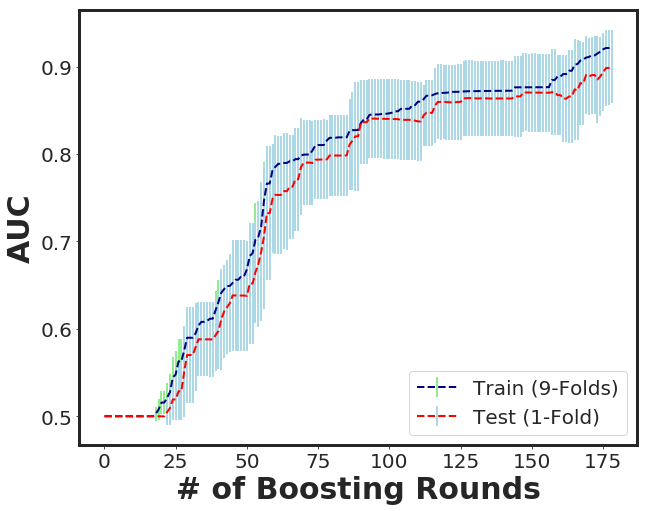_plot_importance(bst, figsize=(10,6), importance_type = "total_gain")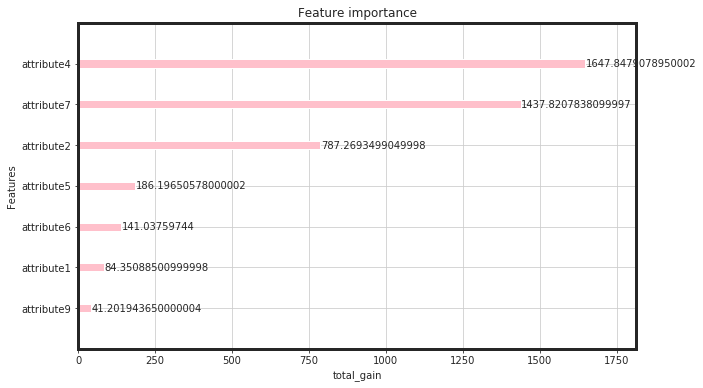Tree_explainer = shap.TreeExplainer(bst)
shap_Treevalues = Tree_explainer.shap_values(df_X)
shap.summary_plot(shap_Treevalues, df_X, plot_type = "bar", color = "pink", max_display = 10)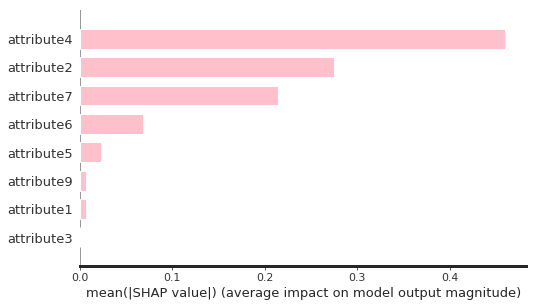### As seen, attribute3 has not played an important role through the feature selection process. Therefore, I dropped it and build the final model based on that.

df_pruned = df_X.drop(["attribute3"], axis = 1)


### As seen, the total gain for attribute4, attirbute7, and attribute 2 are higher than the rest. This would say that, for linear models such as regulirized linear models including elastic net, these features will be pruned. Now, we can see, how these features perform:

_plot_roc_nfolds_xgboost(df_pruned, Y)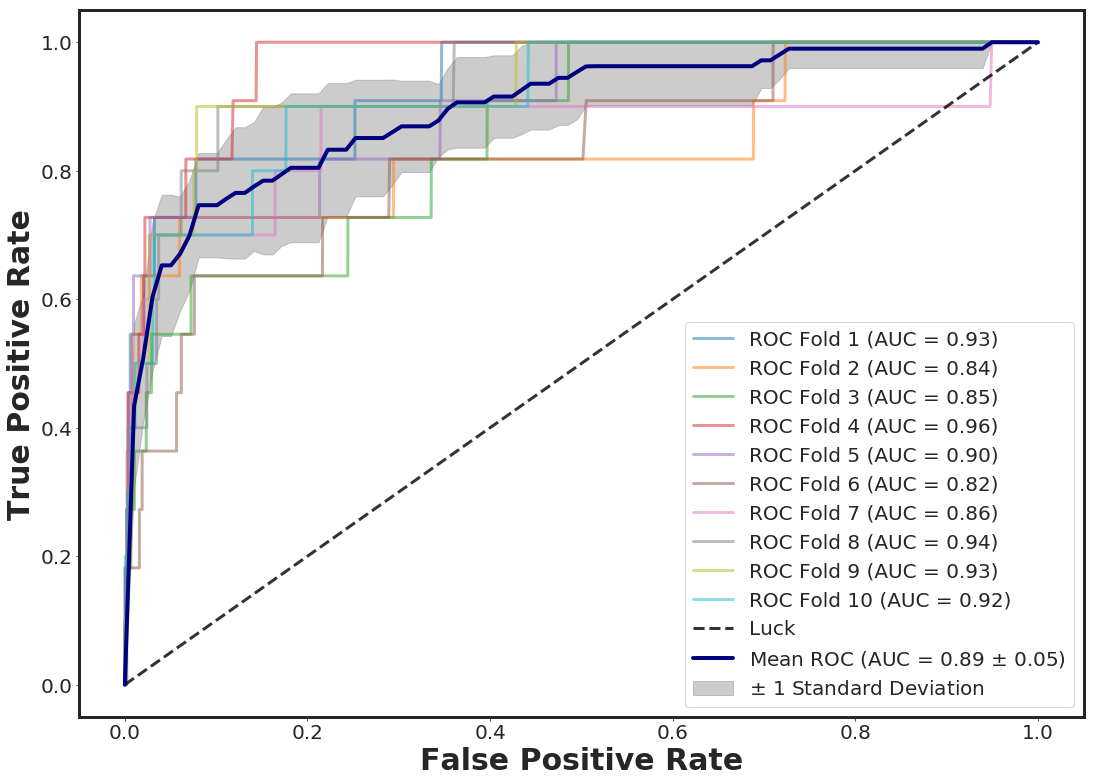test_size = 0.3
n_folds = 10
accuracy_score, roc_score, cv_roc_score, y_pred_prob, X_test, y_test, optimal_threshold = _clf_xgboost(df_pruned, Y, test_size, n_folds)
CI_neg, CI_pos = _ConfidenceInterval(roc_score, X_test.shape)
print(F"Accuracy for test size {test_size}  = {accuracy_score:.5f}")
print(F"ROC for test size {test_size} = {roc_score:.3f}")
print(F"Mean ROC for {n_folds} folds CV = {cv_roc_score.mean():.3f}")
print(F"Confidence Interval Accuracy = [{CI_neg:.3f} , {CI_pos:.3f}]")

class_names = [0, 1]
#optimal threshold based on Youden Index
y_pred = (y_pred_prob[:,1] > optimal_threshold).astype(int)
# Compute confusion matrix
cnf_matrix = confusion_matrix(y_test, y_pred)
np.set_printoptions(precision=2)
# Plot normalized confusion matrix
plt.figure(figsize=(6,6))
_plot_confusion_matrix(cnf_matrix, classes=class_names, normalize = True)
plt.show()

Accuracy for test size 0.3  = 0.99914
ROC for test size 0.3 = 0.890
Mean ROC for 10 folds CV = 0.896
Confidence Interval Accuracy = [0.887 , 0.893]
Normalized confusion matrix
[[0.91 0.09]
[0.25 0.75]]# Discussion:

### As seen, the xgboost approach showed a good performance in terms of classification of the individuals in terms of Failure and Non-failure. The classification problem is an imbalanced problem (prevalence < 1%). Therefore, the classification accuracy by itself cannot be trusted and the other classification metrics such as area under ROC curve and Precision-Recalls or F-1 score which are robust to imbalanced classes should be used. The other challenge is the size of the dataset. In fact, we are looking for a model that can be generalized to a larger population. In this regard, it is also better to report the classification error with a confidence interval at a statistical significance level. For instance, to have 95% significance, we can calculate the classification error from the following formula using the Z-score of 1.96:

$ConfidenceInterval = Score \pm 1.96 \times \sqrt{ \frac{Score \times (1 - Score)}{N}}$

### For instance, for the approach , we have N= 37349 (30% of the data) individuals in the testing set (unseen individuals) with an AUC of 0.89 which is within the calculated 95% confidence interval. Now, we can add the prediction pribabilities for both class 0 and 1 to the test data.

X_test["pred_proba_class_0"] = pd.Series(y_pred_prob[:,0], index = X_test.index)
X_test["pred_proba_class_1"] = pd.Series(y_pred_prob[:,1], index = X_test.index)

print(F"Test Size = {X_test.shape}")

Test Size = (37349, 9)

attribute1 attribute2 attribute4 attribute5 attribute6 attribute7 attribute9 pred_proba_class_0 pred_proba_class_1
22999 0.394775 -0.073170 -0.076004 -0.202137 -0.414557 -0.039335 -0.065047 0.999829 0.000171
26237 -1.097957 0.844409 -0.076004 0.613269 -2.623549 -0.039335 -0.065047 0.997224 0.002776
123435 -1.179217 -0.073170 -0.076004 -0.515755 0.573101 -0.039335 -0.065047 0.999710 0.000290
120834 0.404221 -0.073170 -0.076004 -0.515755 1.461849 -0.039335 -0.065047 0.999801 0.000199
31117 0.243279 -0.073170 -0.076004 1.428676 0.208837 -0.039335 -0.065047 0.999160 0.000840

### Now we can repeat this process for N times (let’s do 100 times) and check the histograms of auc for 95% significance level.

_xgboost_auc_hist(df_pruned, Y, n_iterations = 100, alpha = 0.95)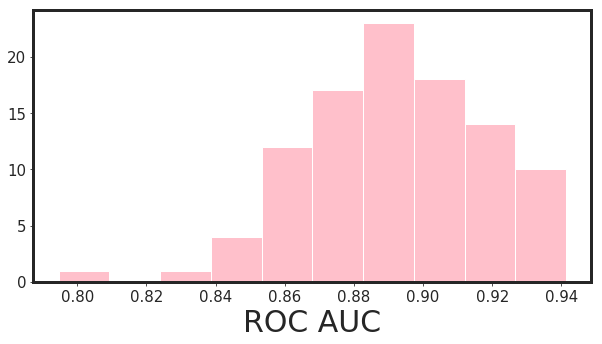95.0% Significance Level - ROC AUC Confidence Interval= [0.849 , 0.934]


# 2nd Approach: Regularized Linear Model

# Ridge
alpha = 0.0

glmnet_model = _glmnet(df_X, Y, alpha = alpha)
_plot_glmnet_coeff_path(glmnet_model, df_X)
_plot_glmnet_cv_score(glmnet_model)
_df_glmnet_coeff_path(glmnet_model, df_X)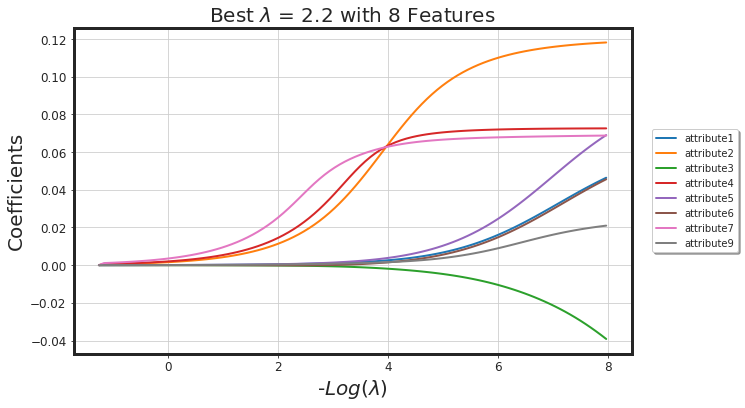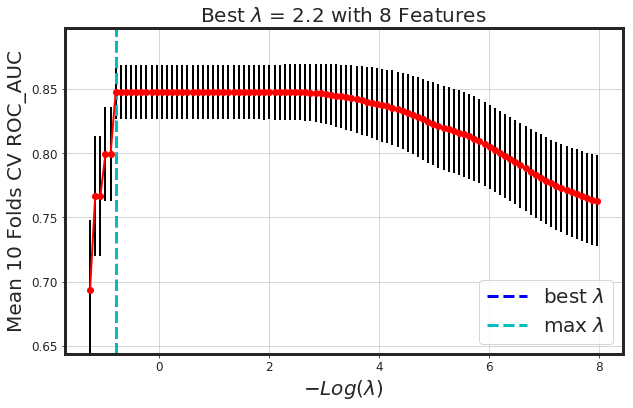Features Coeffs
0 attribute7 0.001592
1 attribute4 0.000901
2 attribute2 0.000707
3 attribute5 0.000030
4 attribute1 0.000027
5 attribute9 0.000022
6 attribute6 -0.000007
7 attribute3 -0.000013
_glmnet_best_score_alpha(df_X, Y)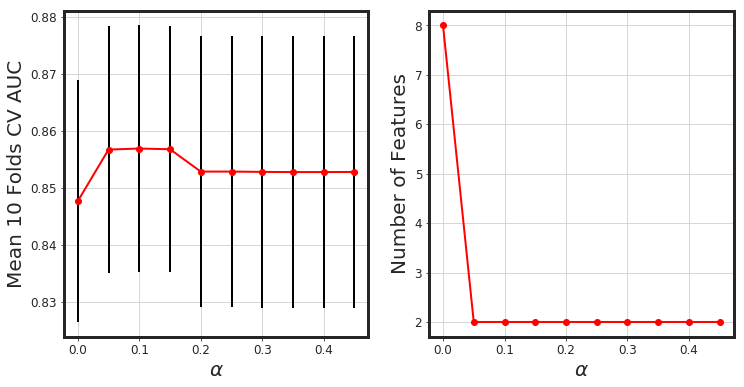# Elastic-Net
alpha = 0.1

glmnet_model = _glmnet(df_X, Y, alpha = alpha)
_plot_glmnet_coeff_path(glmnet_model, df_X)
_plot_glmnet_cv_score(glmnet_model)
_df_glmnet_coeff_path(glmnet_model, df_X)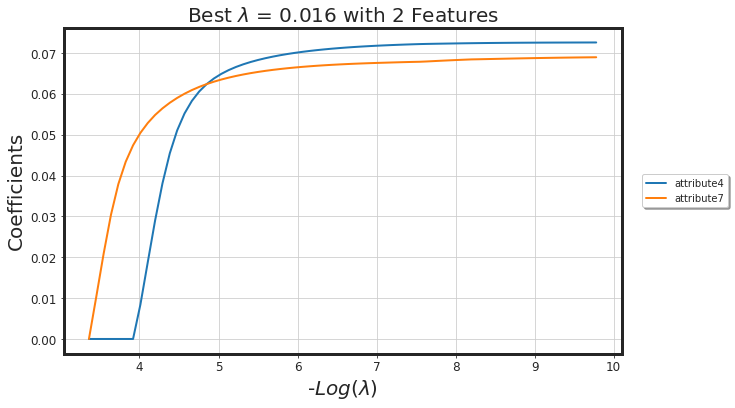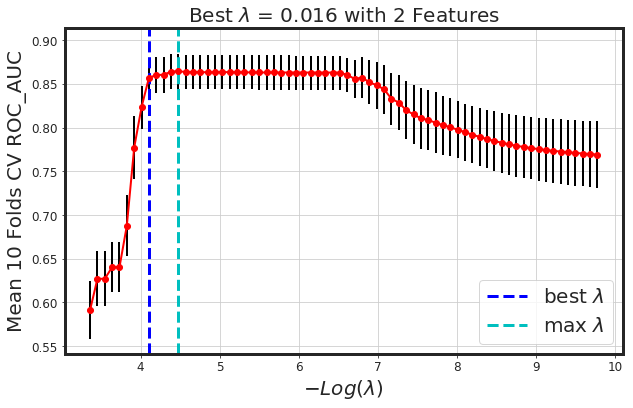Features Coeffs
0 attribute7 0.052871
1 attribute4 0.018768

# Notes could be considered:

### Other than, hyper parameter optimization (using grid search or bayesian optimization), I have not implemented any neural network models here that could be used as well (neural network without hyper-parameter optimizaiton is technically useless). Another thing that could be used is the time at event. For example, any useful information such as some specific day-of-the-month that failure happened can be engineered as a new feature (attribute). In addition to this, the device include 1169 unique values where failure happened for 106 of them.

g = df_raw.groupby("device").agg({"failure" : "mean"}).sort_values(by = ["failure"], ascending = False)
g = g.reset_index(level=["device"])
print(F"Number of groups with non-zero failure rate = {g[g['failure'] > 0].shape}")

Number of groups with non-zero failure rate = 106

device failure
0 S1F0RRB1 0.200000
1 S1F10E6M 0.142857
2 S1F11MB0 0.142857
3 S1F0CTDN 0.142857
4 Z1F1AG5N 0.111111
5 W1F0PNA5 0.111111
6 W1F13SRV 0.076923
7 W1F03DP4 0.071429
8 W1F1230J 0.071429
9 W1F0T034 0.058824